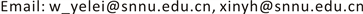1陕西师范大学物理学与信息技术学院，陕西 西安

2西安航空学院机械工程学院，陕西 西安1. 引言

2. 算法描述

2.1. 比特面图像分层原理

1) 将原图像转换为灰度图像并作为第一层图像。

2) 将第一层图像的最低有效比特层置零，得到一幅包含了七个比特层的图像作为第二层图像输出，接着对第二层图像的最低有效比特层置零作为第三层图像输出，以此类推，直到图像仅保留一个比特层。

3) 将得到的比特层数不同的二进制数据转换成十进制像素值，从而获得与比特层数相匹配的多层图像。

2.2. 彩色转换

1) 将原图像作为参考图像Io (original image)，分层后的图像作为待处理图像Ig (gray image)，将Ig扩展到三通道，并将扩展后的图像与Io分别转化到YCbCr空间(其中Y是指亮度分量，Cb指蓝色色度分量，而Cr指红色色度分量)。

2) 对Io构成的图像矩阵的每一列求最大值和最小值，假设图像的分辨率为n ´ m，用 P ( i , j ) n ( 1 < i < n , 1 < j < m ) 来表示第m列的像素点，则矩阵中每一列的最大值和最小值我们可以表示为：

P max m = max ( P 1 , 1 m , P 1 , 2 m , ⋯ , P 1 , n m )

P min m = min ( P 1 , 1 m , P 1 , 2 m , ⋯ , P 1 , n m )

3) 对两幅图像进行归一化处理以得到重构的彩色图像模具  ：

M a r k 1 = I g − I g min I g max − I g min , (1)

M a r k 2 = I o − I o min I o max − I o min , (2)

4) 进行色彩映射转移，将Mark2中的像素值转移到对应的Mark1中的像素点上，使分层后的灰度图像具有与原图像相同的色彩结构。转换结果如图3所示。

3. 利用分层PCA生成显著图

3.1. 提取结构特征

p a = 1 N ∑ i = 1 N     p i (3)

d ( p i , p a ) = 1 N × ∑ i = 1 N     p i × ( i x − a x ) 2 + ( i y − a y ) 2 S ( i , a ) 2 (4)

P ( p i ) = ‖ p ′ i ‖ 1 (5)

3.2. 提取颜色特征

C ( r i ) = ∑ j = 1 M ‖ r i − r j ‖ 2 (6)

3.3. 结构特征与颜色特征的显著性融合

D ( p i ) = P ( p i ) ⋅ C ( p i ) (7)

1) 用融和特征映射D(pi)检测不同层的像素集,计算每个阈值结果的重心。

2) 每个重心放置一个δ设为10,000的高斯分布，计算各个阈值相应的高斯权重。

3) 在各层图像中心处增加一个权重为5的高斯分布，提高中心位置的权重。

S ( p i ) = D ( p i ) ⋅ G ( p i ) (8)

3.4. 最优结果判决

E n s ( X ) = − ∑ i = 1 m   ∑ j = 1 n     p ( X i , j ) log p ( X i , j ) (9)

k o p t = argmin k ( E n s ( S k ) ) ,     k = 1 , ⋯ , K (10)

4. 实验结果及分析

Entropy of hierarchical image informatio

PRE = TP TP + FP (11)

REC = TP TP + FN (12)

FME = 2 × PRE × REC PRE + REC (13)

HS算法在准确率与F-measure值上有一定的优势。总体上，本文算法在不同数据集上的准确率均超过90%，并且平均F-measure值高于其他算法，检测效果稳定，鲁棒性较好。

5. 结论